﻿ 澜沧江允景洪水文站历史洪水水沙关系研究

# 澜沧江允景洪水文站历史洪水水沙关系研究Relationship between Water and Sediment in Historical Floods at Yunjinghong Gauging Station, Lancang River

Abstract: Investigation of historical flooding elements is a key point in hydrological surveys, aiming to explore historical hydrological regime out of the period of hydrological observation and provide data support and scientific basis for the construction and management of water conservancy projects. Based on flood data gauged at Yunjinghong hydrology station, Relationship between water level, discharge and sediment content was detected. Through the analysis and test of correlation, the relationship between water and sediment of the natural river in the flood season in the historical period was restored. Results in this work could be the foundation for recovering the information of historical flood with trace deposits.

1. 引言

2. 研究区域概况

3. 数据与方法

3.1. 数据来源

3.2. 分析方法

$r=\frac{n{\sum }^{\text{​}}xy-{\sum }^{\text{​}}x{\sum }^{\text{​}}y}{\sqrt{\left[n{\sum }^{\text{​}}{x}^{2}-{\left({\sum }^{\text{​}}x\right)}^{2}\right]\left[n{\sum }^{\text{​}}{y}^{2}-{\left({\sum }^{\text{​}}y\right)}^{2}\right]}}$ (1)

r值在+1与−1之间，其值越接近±1，表示两变量直线相关的程度越高，月接近零，则相关程度越低；正号r值表示两变量有同增同减的同方向变动关系，反之为一增一减的异方向变动关系。直线回归分析方法：直线回归的一般方程式是y = a + bx，式中的x是自变量，y是因变量，称y倚x回归方程。在方程中，用最小平方法估计参数b的公式是：

$b=\frac{n{\sum }^{\text{​}}xy-{\sum }^{\text{​}}x{\sum }^{\text{​}}y}{n{\sum }^{\text{​}}{x}^{2}-{\left({\sum }^{\text{​}}x\right)}^{2}}$ (2)

${\sigma }_{y}=\sqrt{\frac{\underset{i=1}{\overset{n}{\sum }}y-{y}_{1}}{n-1}}$ (3)

${s}_{y}=±\sqrt{1-{r}^{2}}$ (4)

4. 结果

4.1. 水位流量关系

4.1.1. 影响因素分析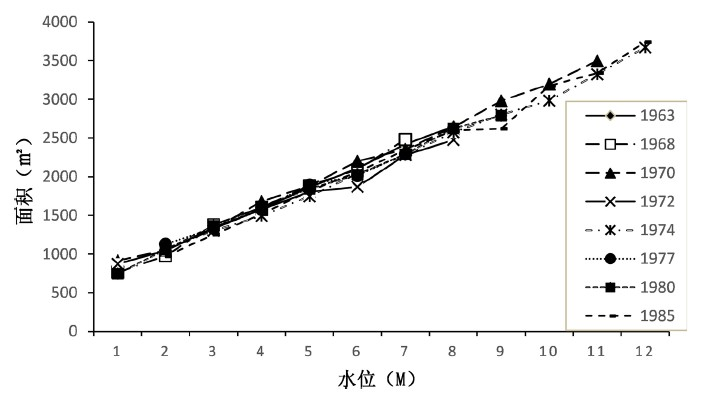Figure 1. Curve of water level-sectional area of Yunjing Flood Station

4.1.2. 趋势分析Table 1. Cross-sectional area of each year under different water levels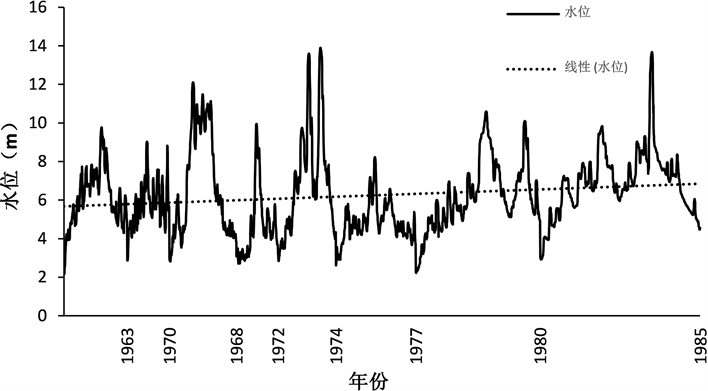Figure 2. Trend of water level over the years

4.1.3. 水位–流量关系分析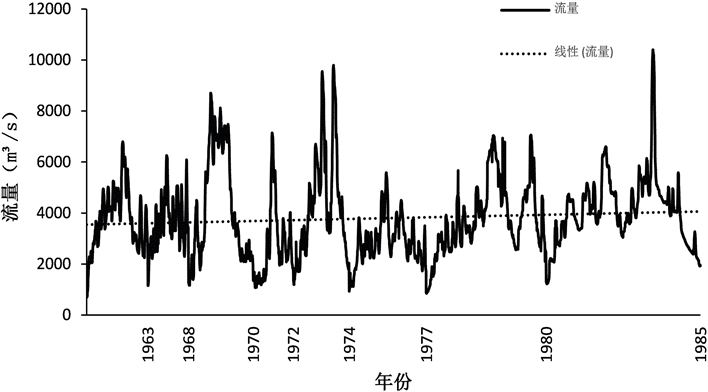Figure 3. Trend of flow over the years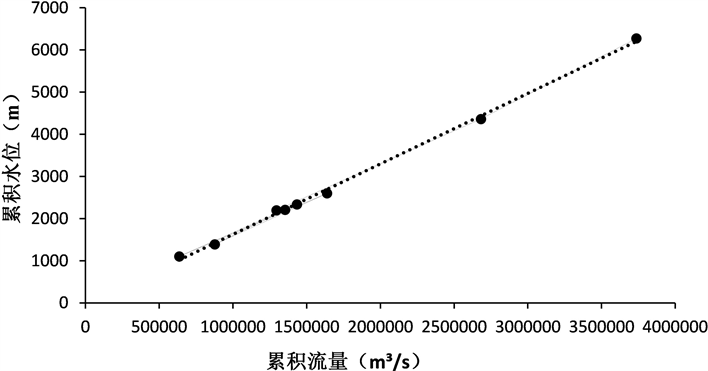Figure 4. Double-accumulation curve of water level flowFigure 5. Relationship between water level and flow rate at each stageTable 2. Regression equations of sediment concentration in each period

4.2. 流量含沙量关系

4.2.1. 趋势分析

4.2.2. 流量-含沙量关系分析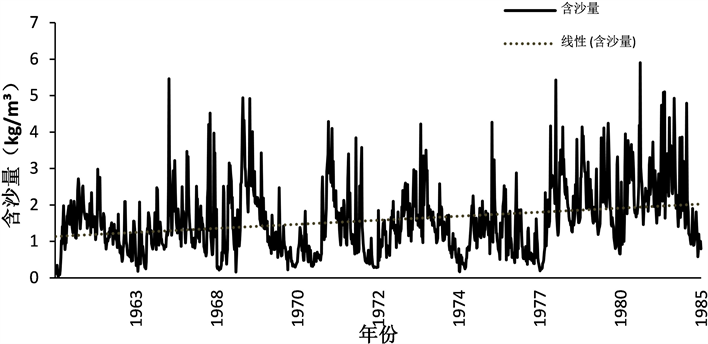Figure 6. Trend of sediment concentration over the years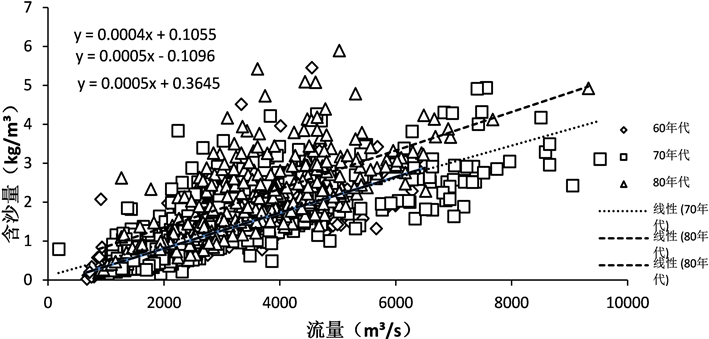Figure 7. Curve of sediment concentration in runoff at each stage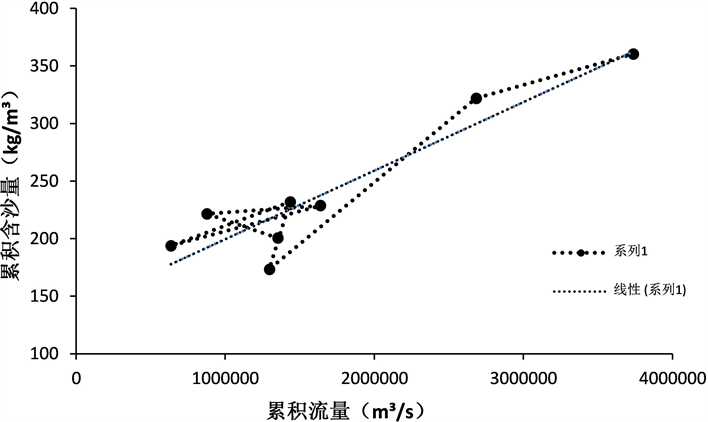Figure 8. Double accumulated curve of sediment concentration

4.2.3. 回归线误差

60年代：0.52；70年代：0.55；80年代：0.75

5. 讨论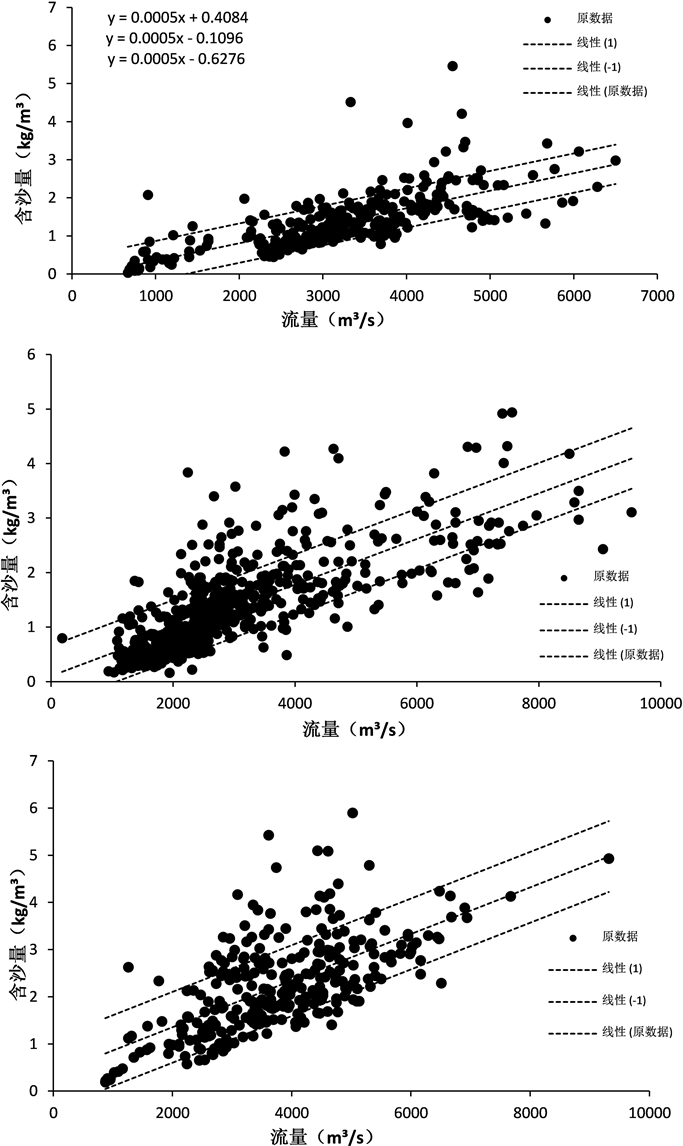Figure 9. Relationship between runoff and sediment concentration in the 60~80 years

6. 结论

 杨玉荣. 宜昌站水文资料的推算和特性分析[J]. 人民长江, 1989(11): 38-44.

 徐德龙, 荣风聪. 大宁河巫溪站历史洪水分析研究[J]. 水利水电快报, 1999(2): 28-29.

 温维超. 大华电站水位流量关系的确定[J]. 云南水力发电, 2002, 18(s1): 25-26.

 李廷华. 景洪水电站历史洪水分析[J]. 云南水力发电, 2001, 17(1): 25-27.

 李万寿, 高小平, 孙胜利. 青海省境内黄河干流水沙量变化历史分析[J]. 水土保持通报, 2001, 21(2): 18-22.

 邹高禄, 罗怀良. 澜沧江河道冲淤变化与来沙系数的格兰吉尔因果分析[J]. 地理科学, 2004, 24(6): 698-703.

 李晓刚, 黄春长, 庞奖励, 等. 汉江上游白河段万年尺度洪水水文学研究[J]. 地理科学, 2012, 32(8): 971-978.

 黄春长, 李晓刚, 庞奖励, 等. 黄河永和关段全新世古洪水研究[J]. 地理学报, 2012, 67(11): 1493-1504.

 詹道江, 谢悦波. 古洪水研究[M]. 北京: 中国水利水电出版社, 2001.

 周正道, 李云霞. 功果桥站水位-流量关系分析[J]. 西安文理学院学报(自然科学版), 2012, 15(1): 107-110.

 周安辉. 澜沧江近20年泥沙变化特性分析[J]. 人民长江, 2012(s1): 114-115.

 王亿春, 胡云萍. 浅析澜沧江上游水利工程对景洪水文站水文要素的影响[C]//云南省水利学会. 2014年度学术交流会论文集: 2014年卷. 城市: 出版社, 2014.

Top